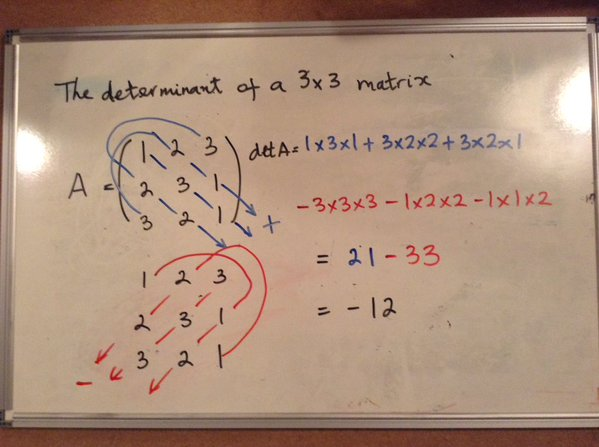Oh, the days – weeks, even – of my university life I spent working out the determinants of matrices. The 3×3 version was the main culprit, of course, usually needing to be split down into three smaller determinants, and usually requiring a sign change in one or two that I’d almost always miss.

A mess, if ever there was one.

Luckily, there’s another method for 3×3 matrices that I find much easier than the old-fashioned way.

We’re going to be looking at diagonal lines in matrices, and there are six of them. There don’t seem to be six, until you realise that the diagonals wrap around. There are three forward diagonals:

• (top left, middle middle, bottom right) is the obvious one;
• (top middle, middle right, bottom left) and
• (top right, middle left, bottom middle)

The three backward ones are:

• (top left, middle right, bottom middle);
• (top middle, middle left, bottom right) and
• (top right, middle middle, bottom left)

Once you recognise the diagonals, the recipe is simple: you’re going to multiply together the numbers on each diagonal to get six products. Add the three forward ones, subtract the three backward ones and boom! There’s your determinant.

For example, let’s take the matrix $\left( \begin{array}{ccc} 1 & 2 & 3 \\ 2 & 3 & 1\\ 3 & 2 & 1 \end{array}\right)$.

The forward diagonals are (1, 3, 1), (2, 1, 3) and (3, 2, 2), giving products of 3, 6 and 12 – these add up to 21.

The backward diagonals are (1, 1, 2), (2, 2, 1) and (3, 3, 3), giving products of 2, 4 and 27, which add up to 33.

$21 - 33 = -12$, which is indeed the determinant of the matrix!

UPDATE: @DrTrapezio, who is Luciano Rila in real life, has very kindly drawn out the calculation as he would do it:Thanks for sharing!

* Edited 2015-12-01 to fix LaTex. Thanks, @fenneklyra, for spotting the error!